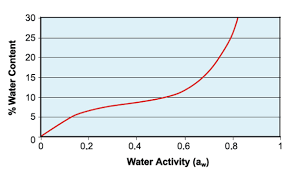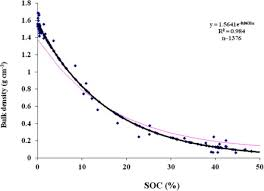## How to Calculate and Solve for Water Content | Soil Mechanics and FoundationThe image above represents water content.

To compute for water content, two essential parameters are needed and these parameters are Mass of the water (Mwand Mass of the solid (Ms).

The formula for calculating water content:

w = Mw / Ms

Where:

w = Water Content
Mw = Mass of the Water
Ms = Mass of the Solid

Let’s solve an example;
Find the water content when the mass of the water is 28 and the mass of the solid is 7.

This implies that;

Mw = Mass of the Water = 28
Ms = Mass of the Solid = 7

w = Mw / Ms
w = 28 / 7
w = 4

Therefore, the water content is 4.

Calculating the Mass of the Water when the Water Content and the Mass of the Solid is Given.

Mw  = w x Ms

Where;

Mw = Mass of the Water
w = Water Content
Ms = Mass of the Solid

Let’s solve an example;
Find the mass of the water when the water content is 15 and mass of the solid is 4.

This implies that;

w = Water Content = 15
Ms = Mass of the Solid = 4

Mw  = w x Ms
Mw  = 15 x 4
Mw  = 60

Therefore, the mass of the water is 60.

## How to Calculate and Solve for Bulk Density | Soil Mechanics and FoundationThe image above represents bulk density.

To compute for bulk density, two essential parameters are needed and these parameters are Mass of the soil (m) and Volume of the soil (V).

The formula for calculating bulk density:

sb = m / V

Where:

sb = Bulk Density
m = Mass of the Soil
V = Volume of the Soil

Let’s solve an example;
Find the bulk density when the mass of the soil is 24 and the volume of the soil is 6.

This implies that;

m = Mass of the Soil = 24
V = Volume of the Soil = 6

sb = m / V
sb = 24 / 6
sb = 4

Therefore, the bulk density is 4.

Calculating the Mass of the Soil when the Bulk Density and the Volume of the Soil is Given.

m = sb x V

Where;

m = Mass of the Soil
sb = Bulk Density
V = Volume of the Soil

Let’s solve an example;
Find the mass of the soil when the bulk density is 10 and the volume of the soil is 4.

This implies that;

sb = Bulk Density = 10
V = Volume of the Soil = 4

m = sb x V
m = 10 x 4
m = 40

Therefore, the mass of the soil  is 40.